### RANDARRAY Function Examples – Excel & Google Sheets

This tutorial demonstrates how to use the RANDARRAY Function in Excel to generate an array of random numbers.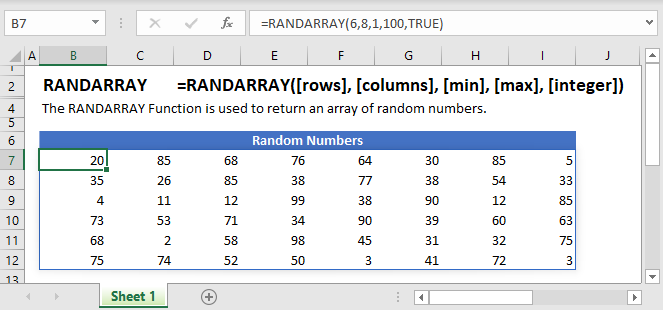## RANDARRAY Function Overview

The RANDARRAY Function is used to return an array of random numbers. It was introduced as a replacement for the RAND Function and the RANDBETWEEN Function.

The RANDARRAY Function simplifies the process of generating random numbers by:

1. Allowing the user to input the formula in only one cell.
2. Allowing the user to specify the number of random values to be generated.

To use the RANDARRAY Excel Worksheet Function, select a cell and type: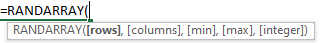(Notice how the formula inputs appear)

## RANDARRAY function Syntax and inputs:

`=RANDARRAY([rows], [columns], [min], [max], [integer])`

[rows] (optional)  – The number of rows to be returned and when left blank it defaults to 1.

[columns] (optional)  – The number of columns to be returned and when left blank it defaults to 1.

[min]  (optional) – The minimum number you would like returned and if left blank it defaults to 0. When a value is specified for this input it should be less than the value provided for the [max] argument.

[max] (optional) – The maximum number you would like returned and if left blank it defaults to 1. When a value is specified for this input it should be greater than the value provided for the [min] argument. You should note that when both the [min]  and [max] functions are left blank the RANDARRAY Function returns an array of numbers between 0 and 1.

[integer] (optional) – Return a whole number or a decimal value. You can enter either TRUE to return a whole number or FALSE to return a decimal number. When this argument is left blank it defaults to FALSE therefore the formula returns a decimal number.

# How to use the RANDARRAY Function

AutoMacro - VBA Code Generator

## To return multiple rows or columns

We can change the size of the array that the RANDARRAY Function returns by changing the number of rows and columns.  To do this we specify the [rows] and [columns] arguments.

To return an array of random numbers that is 5 Rows tall and 1 Column wide, we enter the following formula in cell A2:

`=RANDARRAY(5, 1, 1, 10, TRUE)`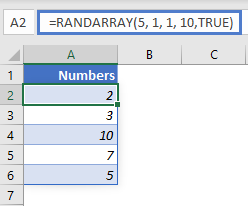To return an array of random numbers that is 5 Rows tall and 3 Columns wide, we enter the following formula in A2:

`=RANDARRAY(5, 3, 1, 10, TRUE)`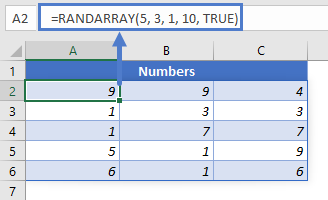## To return numbers between two values

When we want to return numbers between two values we specify the values in the [min] and [max] arguments.

To return a list of random numbers between 1 and 10, we enter the following formula in A2:

`=RANDARRAY(5, 1, 1, 10)`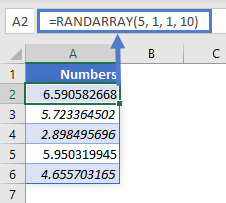## To return  decimal numbers or whole numbers

To return 5 decimal numbers between 1 and 10, we enter the following formula in A2:

`=RANDARRAY(5, 1, 1, 10, FALSE)`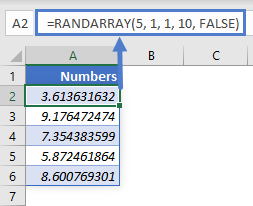Note that we’ve set the [integer] argument to FALSE which means the output will be decimal numbers.

The formula can also be written as:

`=RANDARRAY(5, 1, 1, 10)`

When the [integer] argument is not specified it defaults to FALSE: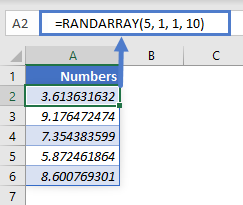To return 5 whole numbers between 1 and 10, we enter the following formula in A2:

`=RANDARRAY(5, 1, 1, 10, TRUE)`Note that we’ve set the [integer] argument to TRUE which means the output will be whole numbers

## Issues

### #VALUE!

This error occurs when the value in the [min] argument is greater than the value in the [max] argument.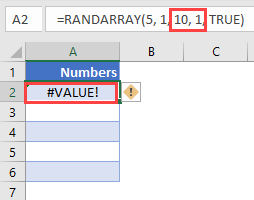To correct this error, set the value in the  [min] argument to a value that is less than that in the [max] argument.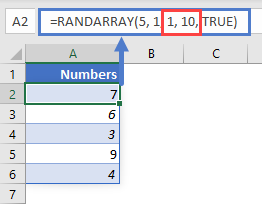## #SPILL!

This error occurs when there is a value in the Spill Range i.e. the range where the RANDARRAY Function places its results.

To correct this error, clear the range that Excel highlights.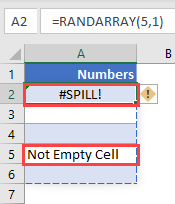## RANDARRAY Tips & Tricks

1. Since all the arguments in the RANDARRAY Function are optional, entering the formula below in any cell returns a single value between 0 and 1:

`=RANDARRAY()`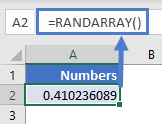2. If the [min] and [max] arguments are not specified, RANDARRAY will default to 0 and 1 respectively and return an array of random numbers between 0 and 1.
`=RANDARRAY(5, 1, , , FALSE)`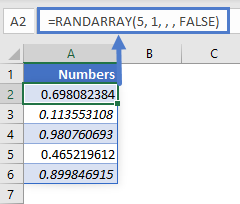3. If the [integer] argument is not specified, RANDARRY will default to FALSE and return an array of decimal numbers:
`=RANDARRAY(5, 1, 1, 10 )`4. RANDARRAY is different from the RAND and RANDBETWEEN functions. While RAND and RANDBETWEEN return a single value, RANDARRAY returns an array.
5. Ensure that the cells below the input cell are blank to avoid the Spill Error.

## RANDARRAY in Google Sheets

The RANDARRAY Function works exactly the same in Google Sheets as in Excel: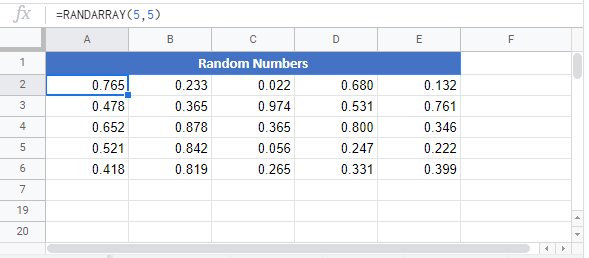## Excel Practice Worksheet

Practice Excel functions and formulas with our 100% free practice worksheets!

• Automatically Graded Exercises
• Learn Excel, Inside Excel!